# 15 In Binary

In this mini-lesson, we will learn about writing 15 in binary along with examples.

Language is a medium to communicate with each other or to express our feelings. Even computers have their own language. Computers understand only 0's and 1's. They are called bits. A computer always works with numbers. Be it a word, image, audio, or video, everything is converted into 0's and 1's.

In math, we follow a standard system to represent numbers. We call it the number system.

We have four different types of number systems with different bases. We can write any digit in any of these number systems.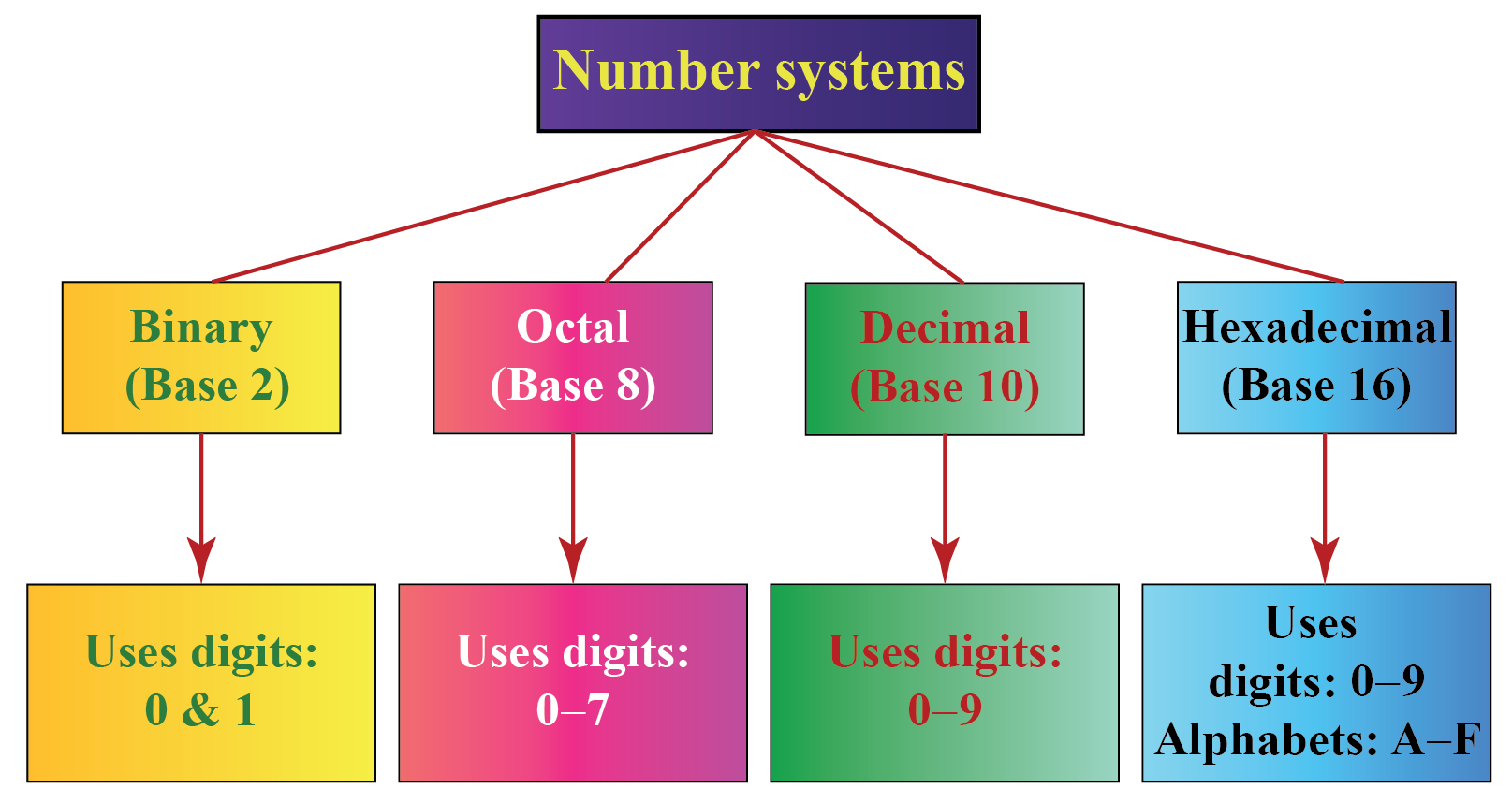Let us learn how to write 15 in binary on this page.

## What Is Meant by Converting 15 From Decimal to Binary?

In our daily life, we represent numbers using the digits 0, 1, 2, ..., 9. This system where we use the digits 0, 1, 2, ..., 9 to write numbers is called the "decimal system."

Though we do not write any base to the numbers we write in our daily life, the default base is 10. That is, we follow the base 10 number system.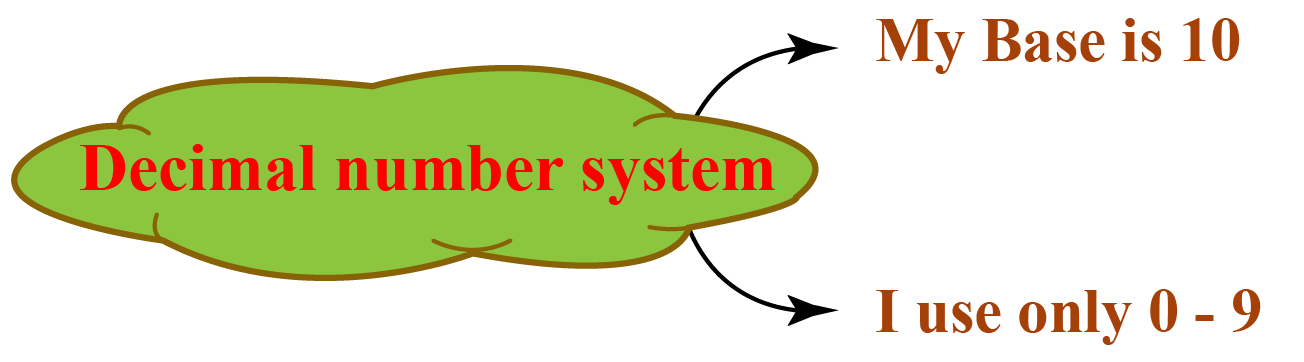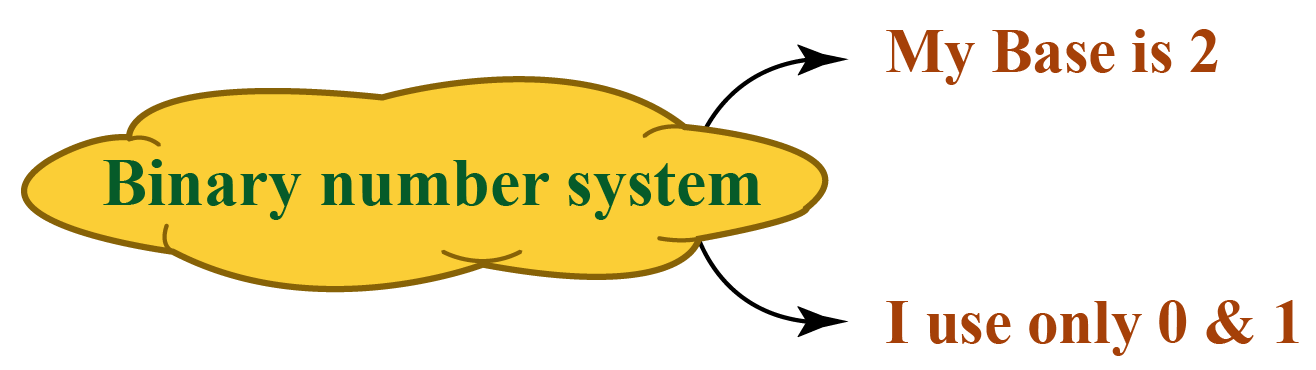Therefore, 15 can also be written as $$15_{10}$$ in a decimal representation.

Converting 15 from decimal to binary means to write or represent 15 using 2 bits only, i.e., 0 and 1 because the base-2 number system uses only 2 digits (0 and 1).

$$15_{10}$$ in binary is $$1111_{2}$$.

## How to Convert 15 From Decimal to Binary Number?

Here, 15 has no base. So by default, its base is 10

The base of the binary system is 2

So we have to convert $$15_{10}$$ to a number with base 2 say $$X_{2}$$.

To convert 15 into binary, we have to divide 15 by 2 and note down the quotient and the remainder in the “quotient-remainder” form.

Repeat this process (dividing the quotient again by 2) until we get the quotient to be less than the base.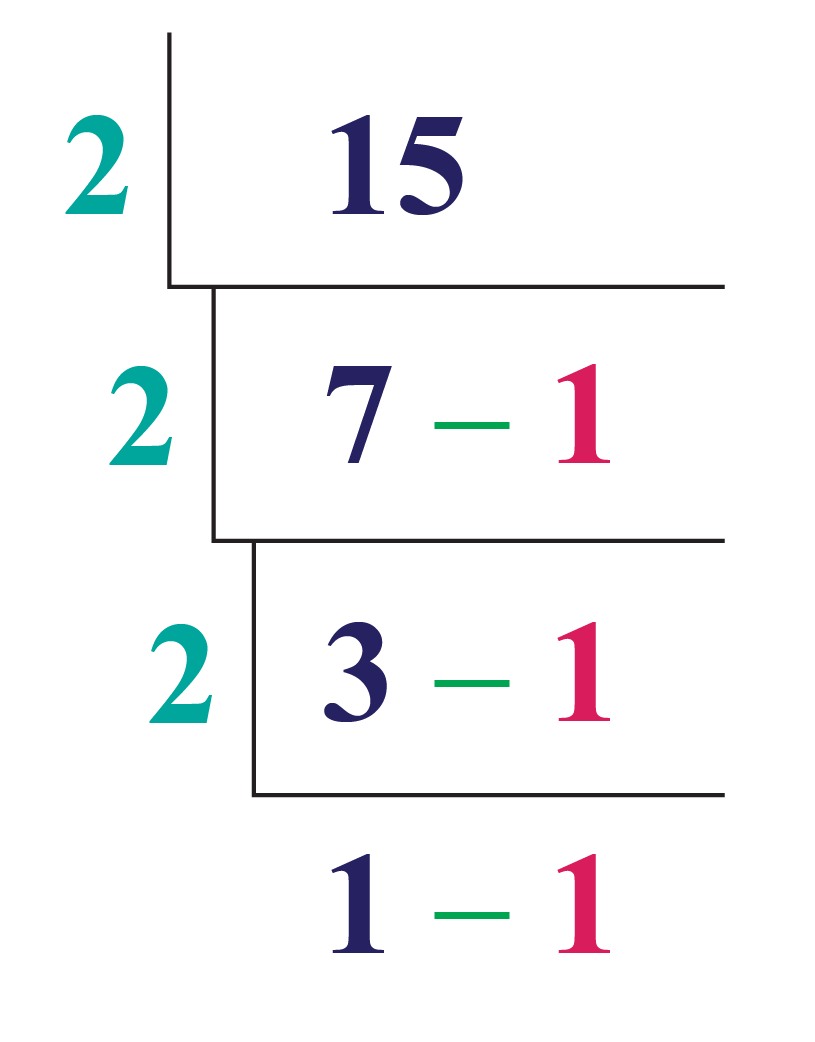15 in the binary system is obtained just by noting down all the remainders and the last quotient from bottom to top.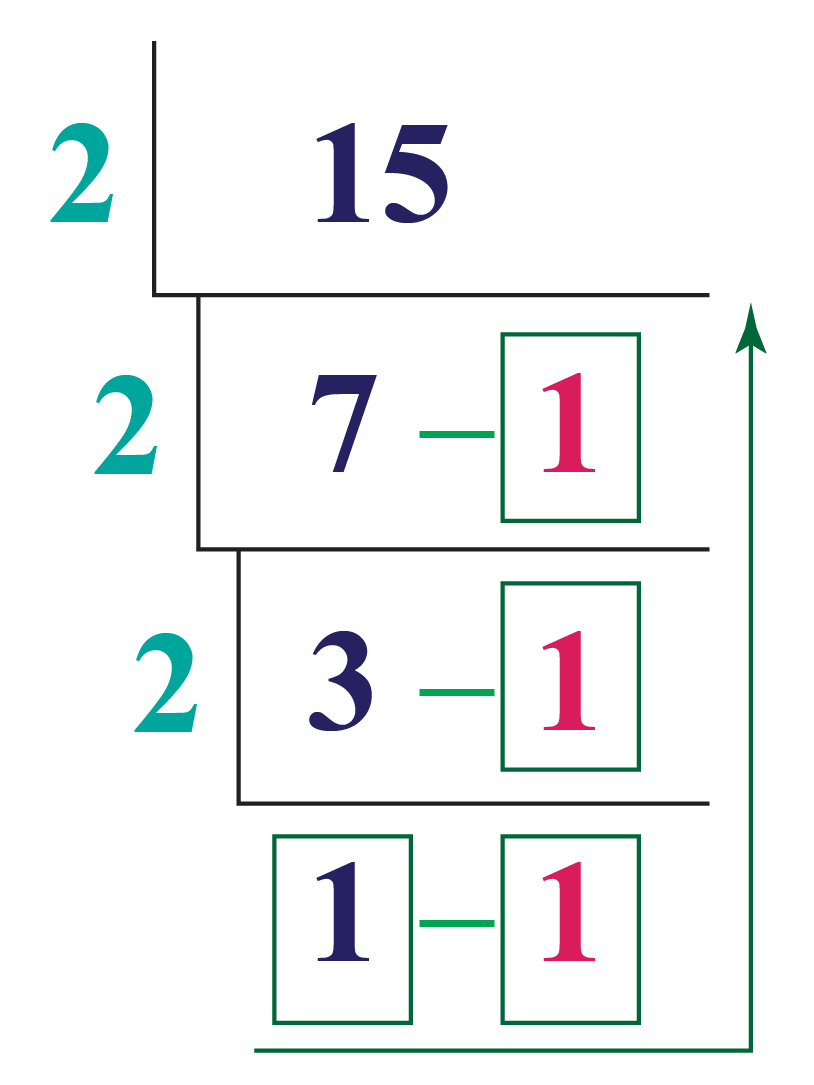Thus, 15 in binary is:

 15 (or) $$15_{10}$$ = $$1111_{2}$$

You can convert any number in a decimal system to a binary-based number using the following calculator:

• Sometimes the base is also referred to as "Radix."
• Base-2 number system uses only 2 digits (0 and 1), base-8 number system uses 8 digits (0-7), base-10 number system uses digits from (0-9), and base-10 number system uses digits from (0-9) and alphabets (A-F).

More Important Topics
Numbers
Algebra
Geometry
Measurement
Money
Data
Trigonometry
Calculus